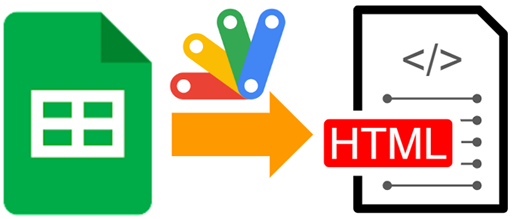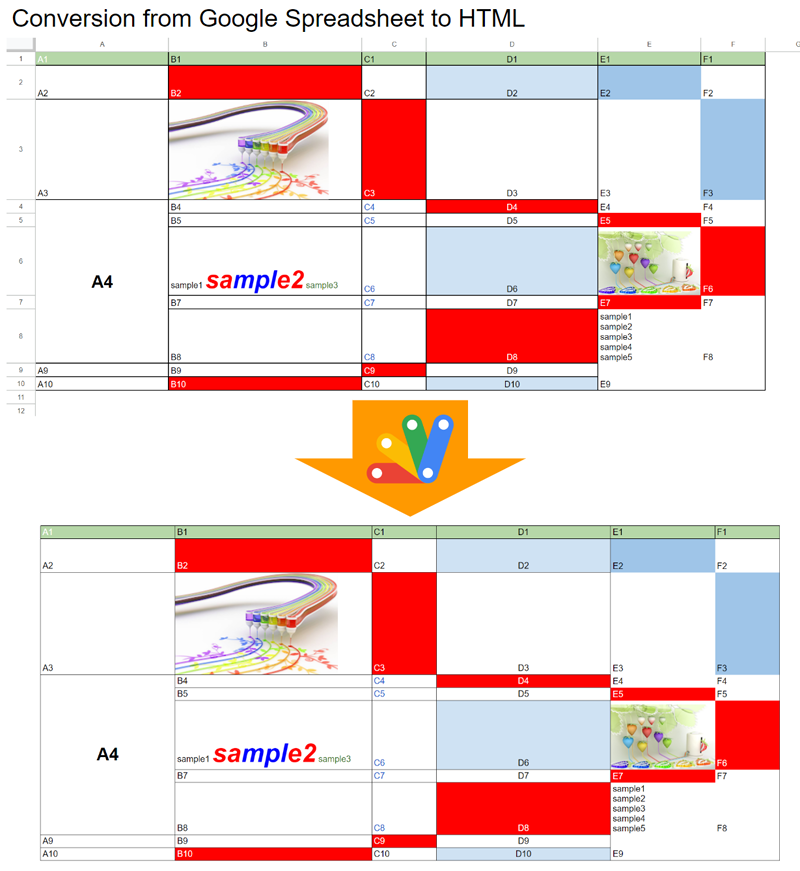GistsThis is a sample script for converting Google Spreadsheet to an HTML table using Google Apps Script.

There is the case that it is required to convert a sheet in a Google Spreadsheet to an HTML table. For example, there might be a situation that a sheet in a Google Spreadsheet is sent as an email including an HTML table. And, there might be a situation in which a sheet in a Google Spreadsheet is published to an HTML page including the converted HTML table. I have published the method for achieving this before. Ref But, in that case, the column width, the row height, merged cells, and the images in the cells couldn’t be used. When those are included in the script, the script becomes complicated. So, I couldn’t include it. But, recently, I have come up with a simple method for achieving this. In this post. I would like to introduce a sample script for converting a sheet in a Google Spreadsheet to HTML.

## Flow

The flow of this method is as follows.

1. Create a temporal sheet.
2. Copy the data range from the source sheet you want to convert to a temporal sheet.
• In the current stage, when Google Spreadsheet is exported as ZIP data, the ZIP file includes HTML data converted from Google Spreadsheet.
• This flow can process without creating the ZIP data as a file.
4. Retrieve the HTML data of the temporal sheet from the ZIP data.
5. Process the header row and column of the HTML data.
6. Create the HTML as an HTML file.

## Sample script

Please copy and paste the following script to the script editor of Google Spreadsheet and set your sheet name and save the script.

/**
* ### Description
*
* @param {String} srcSheetName Source sheet name.
* @return {String} Converted HTML table.
*/
const srcSheet = ss.getSheetByName(srcSheetName);
const tempSheet = ss.insertSheet("temp");
srcSheet.getDataRange().copyTo(tempSheet.getRange("A1"));
const dataRange = srcSheet.getDataRange();
[...Array(dataRange.getNumColumns())].forEach((_, i) => {
const colWidth = srcSheet.getColumnWidth(i + 1);
tempSheet.setColumnWidth(i + 1, colWidth || 100);
});
[...Array(dataRange.getNumRows())].forEach((_, i) => {
const rowHeight = srcSheet.getRowHeight(i + 1);
tempSheet.setRowHeightsForced(i + 1, 1, rowHeight || 21);
});
tempSheet
.insertRowBefore(1)
.insertColumnBefore(1)
.setRowHeight(1, 1)
.setColumnWidth(1, 1)
.getRangeList(["1:1", "A:A"])
.clear();
const url = https://docs.google.com/spreadsheets/export?exportFormat=zip&id=${ss.getId()}; const blob = UrlFetchApp.fetch(url, { headers: { authorization: "Bearer " + ScriptApp.getOAuthToken() }, }).getBlob(); const blobs = Utilities.unzip(blob); const html = blobs.find((b) => b.getName() == "temp.html"); if (!html) { throw new Error(No${sheetName}.html in zip file.);
}
ss.deleteSheet(tempSheet);
const htmlText = html
.getDataAsString()
.replace(
)
return htmlText;
}

function main() {

// Create HTML as an HTML file.
DriveApp.createFile("sample.html", html);
}


## Testing

When the function main is run, the following result is obtained. You can see that the converted HTML table reflects not only cell and text format, but also the column width, the row height, merged cells, and the images in the cells.Credit: The images in the cells are from http://k3-studio.deviantart.com/

## Note

• In this sample script, the data range is used. If you want to use the specific range, please modify it as follows.

• From

srcSheet.getDataRange().copyTo(tempSheet.getRange("A1"));

• To: For example, when you want to convert the range of “B5:F10” on srcSheet, please modify it as follows.

srcSheet.getRange("B5:F10").copyTo(tempSheet.getRange("A1"));

• When this method is used, I believe that the converted HTML data can be also converted to a table in a Google Document. The sample script is as follows. In this case, please modify the function main as follows. By the way, in this script, please enable Drive API at Advanced Google services.

function main() {

// Create HTML as a Google Document file.
const blob = Utilities.newBlob(html, MimeType.HTML);
const newDocId = Drive.Files.insert(
{ title: "sample", mimeType: MimeType.GOOGLE_DOCS },
blob
).id;
const newDoc = DocumentApp.openById(newDocId);
const t = newDoc.getBody().getTables();
for (let r = 0; r < t.getNumRows(); r++) {
const row = t.getRow(r);
[2, 1].forEach((e) => {
const width = row.getCell(e).getWidth();
row.removeCell(e - 1);
row.getCell(e - 1).setWidth(width);
});
}
[...Array(2)].forEach((_) => t.removeRow(0));
}

• In this script, when the size of the data range in Spreadsheet is larger than that of the page size in Google Document, it seems that the table size is forcibly fixed to the page size of Google Document. Please be careful about this.